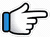# Faded – Alan Walker – Keyboard NotesNew Notes

## Faded – Alan Walker Keyboard Notes

For Faded – Alan Walker Notes as Western or Indian Notations, Click –

Western – CDE FGAB

Indian – srg mpdn

Hindi – सारेग मपधनि

## Keyboard Notes for Faded – Alan Walker – Indian Notation (srg mpdn)

CDE FGAB

सारेग मपधनि

Keyboard Notes for Faded are given from 2 scales
(based on the starting note for the song).

Version 1: starting from  (original scale)
Version 1: starting from  (mostly white keys, so easy to play)

For both versions,
keyboard notes are written first in easy to understand format,
then full keyboard notes are given for the song Faded.

## Faded Keyboard Notes 1 (from M)

M~ M~ M~ N~~
G*~ G*~ G*~ R*~~
N~ N~ N~ N~
m~ m~ m~ G~~

### Part 1 – 1

to my light
Did you feel us?

Another star

M      M       G   M G

M   D    N~~
to   my  light

M    M    R~  N~~
Did you  feel   us~?

M M M    m~~
Another  star,

m      m G   M~

### Part 1 – 2

Afraid our aim is
out of sight
Wanna see us

Alight

M M     G    M   G
Afraid  our  aim  is

M   D   N~
out of  sight

M M       R*    N~~
Wanna  see  us~~

M D~~~
alight~~
Or
M MD~~

### Part 2

Where are you now?
Where are you now?
Where are you now?

Was it all in my fantasy?
Where are you now?
Were you only imaginary?

N           N   N      N
Where  are you  now?

M M N G* G* R*
N N N N
m m m

N           N    N     N
Where  are  you  now?

N           N   N     N
Where  are you  now?

Some notes are given in bracket,
play those notes fast.

(MMM)         D  D    N D M
Was_it_all   in  my  fantasy?
Or
(MDD)        D N D  MM

N           N     N    N
Where  are  you  now?

(MMM)         (MM) m m m m
Were_you   only_  imaginary?

### Part 3 – 1

Where are you now?
Atlantis
Under the sea
Under the sea

Where are you now?
Another dream

The monster’s running
wild inside of me

N           N     N    N
Where  are  you  now?

M G R
Atlantis

RR*     R*   N
Under the   sea,
Or
RR*    N   N

N           N     N    N
Where  are  you  now?

MMG       n
Another   dream
Or
MGG       n

R*     NR*          NR*
The  monster’s  running

N       R*N     D   n
wild   inside  of  me

G     n~~ N

G    n~~ N

M    m~~
So  lost,
Or
G  G~~

G    n~~ N,

G     n~~ R*

G G~      m DmM

### Part 4

These shallow waters
never met

what I needed

I’m letting go a deeper dive

Eternal silence of the sea,
I’m breathing

Alive

M          MG       MG
These  shallow  waters

MD      N
never  met

M       M   R~ N~~
what  I     neede~~d

MM   Mm     m
I’m   letting  go,

m   mG       M
a   deeper   dive

MMG      MG       M D N
Eternal   silence  of the sea

MM    R*~ N
I’m    breathing,

M  MD
alive

### Part 5

Where are you now?
Where are you now?

Under the bright

You’ve set my heart on fire

Where are you now?
Where are you now?

N           N   N   N
Where  are  you  now?

N           N   N   N
Where are  you  now?

MR*    R*   N
Under the   bright

M      R*R*   N

M      R*  R*  D        M   DM
You  set my heart   on   fire

M           R*  R*   N
Where  are  you now?

M          R*  R*   N
Where  are   you   now?

### Part 6

Repeat Part 3 – 2

Where are you now?
Atlantis
Under the sea
Under the sea

Where are you now?
Another dream
The monster’s running
wild inside of me

N           N     N    N
Where  are  you now?

M G R
Atlantis

RR*     R*   N
Under  the  sea,
Or
RR*    N   N

R R*    R*  N D
under  the sea

N           N     N    N
Where  are  you   now?

MMG      n
Another  dream
Or
MGG    n

R*    NR*           NR*
The monster’s   running

N       R*N     D   n
wild   inside  of   me

G n N

G n N

M     m~~  G   n~~ N,

G     n~~ R*

G G~      m DmM

Now, full Keyboard notes for Faded from M.

## Full Keyboard Notes for Faded (from M)

Starting Music

M~ M~ M~ N~~  |  G*~ G*~ G*~ R*~~  |  N~ N~ N~ N~  |  m~ m~ m~ G~~

Part 1

M  M  G  M G  M  D  N~~  |  M  M  R~  N~~  |  M M M  m~~  |  m  m G  M~
You were the shadow to my light  |  Did you feel us?  |  Another star  |  You fade away

M M  G  M  G M  D  N~  |  M M  R*  N~~  |  M D~~~
Afraid our aim is out of sight  |  Wanna see us  |  Alight

Part 2

Some notes are given in bracket, play those notes fast.

N  N  N  N  |  M M N G* G* R* N N N N m m m  |  N  N  N  N  |  N  N  N  N
Where are you now?  |  Where are you now?  |  Where are you now?

(MMM)  D  D  N D M  |  N  N  N  N  |  (MMM)  (MM) m m m m
Was it all in my fantasy?  |  Where are you now?  |  Were you only imaginary?

Part 3

N  N  N  N  |  M G R  |  RR*  R*  N
Where are you now?  |  Atlantis  |  Under the sea  |  Under the sea

N  N  N  N  |  MMG  n  |  R*  NR*  NR* N  R*N  D  n
Where are you now?  |  Another dream  |  The monster’s running wild inside of me

G  n~~ N  |  G  n~~ N  |  M  m~~  G  n~~ N  |  G  n~~ R*  |  G G~  m DmM

Part 4

M  MG  MG  MD  N  |  M  M  R~ N~~  |  MM  Mm  m  m  mG  M
These shallow waters never met  |  what I needed  |  I’m letting go a deeper dive

MMG  MG  M D N  |  MM  R*~ N  |  M  MD
Eternal silence of the sea,  |  I’m breathing  |  Alive

Part 5

N  N  N  N  |  N  N  N  N
Where are you now?  |  Where are you now?

MR*  R*  N    |  M  R*R* N  |  M  R*  R*  D  M  DM
Under the bright  |  but faded lights  |  You’ve set my heart on fire

M  R*  R*  N  |  M  R*  R*  N
Where are you now?  |  Where are you now?

Part 6

Repeat Part 3 – 2

N  N  N  N  |  MMG  n  |  R*  NR*  NR* N  R*N  D  n
Where are you now?  |  Another dream  |  The monster’s running wild inside of me

G  n~~ N  |  G  n~~ N  |  M  m~~  G  n~~ N  |  G  n~~ R*  |  G G~  m DmM

## Faded Keyboard Notes 2 (from p)

p~ p~ p~ n~~
g*~ g*~ g*~ r*~~
n~ n~ n~ n~
M~ M~ M~ g~~

### Part 1 – 1

to my light
Did you feel us?

Another star

p        p       g   p g

p   d    n~~
to  my  light

p    p     r~   n~~
Did you  feel  us~?

p p p       M~~
Another  star,

M      M g   p~

### Part 1 – 2

Afraid our aim is
out of sight
Wanna see us

Alight

p p       g      p   g
Afraid  our  aim  is

p      d   n~
out  of  sight

p p          r*    n~~
Wanna  see  us~~

p d~~~
alight~~
Or
p pd~~

### Part 2

Where are you now?
Where are you now?
Where are you now?

Was it all in my fantasy?
Where are you now?
Were you only imaginary?

n            n    n     n
Where  are you  now?

p p n g* g* r*
n n n n
M M M

n            n    n    n
Where  are you  now?

n            n    n     n
Where  are you  now?

Some notes are given in bracket,
play those notes fast.

(ppp)             d  d     n d p
Was_it_all   in  my  fantasy?
Or
(pdd)             d n     d  pp

n           n     n    n
Where  are  you  now?

(ppp)          (pp) M M M M
Were_you  only_  imaginary?

### Part 3 – 1

Where are you now?
Atlantis
Under the sea
Under the sea

Where are you now?
Another dream

The monster’s running
wild inside of me

n            n     n     n
Where  are you  now?

p g r
Atlantis

rr*         r*   n
Under  the  sea,
Or
rr*     n   n

n           n     n    n
Where  are  you now?

ppg         s*
Another  dream
Or
pgg         s*

r*     nr*             nr*
The  monster’s  running

n       r*n        d   s*
wild   inside   of  me

g      s* ~~ n

g      s* ~~ n

p      M~~
So   lost,
Or
g    g~~

g     s* ~~ n,

g     s* ~~ r*

g g~       M dMp

### Part 4

These shallow waters
never met

what I needed

I’m letting go a deeper dive

Eternal silence of the sea,
I’m breathing

Alive

p             pg        pg
These   shallow   waters

pd        n
never   met

p        p    r~ n~~
what  I    neede~~d

pp     pM     M
I’m    letting  go,

M   Mg         p
a   deeper   dive

ppg         pg         p d n
Eternal   silence  of the sea

pp    r*~ n
I’m   breathing,

p  pd
alive

### Part 5

Where are you now?
Where are you now?

Under the bright

You’ve set my heart on fire

Where are you now?
Where are you now?

n            n     n     n
Where  are  you  now?

n           n     n     n
Where are  you  now?

pr*        r*     n
Under   the   bright

p       r*r*    n

p        r*     r*   d        p     dp
You  set   my heart  on   fire

p             r*    r*   n
Where  are   you  now?

p           r*  r*    n
Where  are you   now?

### Part 6

Repeat Part 3 – 2

Where are you now?
Atlantis
Under the sea
Under the sea

Where are you now?
Another dream
The monster’s running
wild inside of me

n            n      n    n
Where  are  you now?

p g r
Atlantis

rr*      r*    n
Under   the   sea,
Or
rr*     n   n

r r*  r*  n d
under     the   sea

n           n     n    n
Where  are  you now?

ppg       s*
Another  dream
Or
pgg       s*

r*      nr*           nr*
The   monster’s   running

n       r*n      d   s*
wild   inside  of   me

g s*  n

g s*  n

p     M~~  g   s* ~~ n,

g      s* ~~ r*

g g~        M dMp

Now, full Keyboard notes for Faded from p.

## Full Keyboard Notes for Faded (from p)

Starting Music

p~ p~ p~ n~~  |  g*~ g*~ g*~ r*~~  |  n~ n~ n~ n~  |  M~ M~ M~ g~~

Part 1

p  p  g  p g  p  d  n~~  |  p  p  r~  n~~  |  p p p  M~~  |  M  M g  p~
You were the shadow to my light  |  Did you feel us?  |  Another star  |  You fade away

p p  g  p  g p  d  n~  |  p p  r*  n~~  |  p d~~~
Afraid our aim is out of sight  |  Wanna see us  |  Alight

Part 2

Some notes are given in bracket, play those notes fast.

n  n  n  n  |  p p n g* g* r* n n n n M M M  |  n  n  n  n  |  n  n  n  n
Where are you now?  |  Where are you now?  |  Where are you now?

(ppp)  d  d  n d p  |  n  n  n  n  |  (ppp)  (pp) M M M M
Was it all in my fantasy?  |  Where are you now?  |  Were you only imaginary?

Part 3

n  n  n  n  |  p g r  |  rr*  r*  n
Where are you now?  |  Atlantis  |  Under the sea  |  Under the sea

n  n  n  n  |  ppg  s*   |  r*  nr*  nr* n  r*n  d  s*
Where are you now?  |  Another dream  |  The monster’s running wild inside of me

g  s* ~~ n  |  g  s* ~~ n  |  p  M~~  g  s* ~~ n  |  g  s* ~~ r*  |  g g~  M dMp

Part 4

p  pg  pg  pd  n  |  p  p  r~ n~~  |  pp  pM  M  M  Mg  p
These shallow waters never met  |  what I needed  |  I’m letting go a deeper dive

ppg  pg  p d n  |  pp  r*~ n  |  p  pd
Eternal silence of the sea,  |  I’m breathing  |  Alive

Part 5

n  n  n  n  |  n  n  n  n
Where are you now?  |  Where are you now?

pr*  r*  n    |  p  r*r* n  |  p  r*  r*  d  p  dp
Under the bright  |  but faded lights  |  You’ve set my heart on fire

p  r*  r*  n  |  p  r*  r*  n
Where are you now?  |  Where are you now?

Part 6

Repeat Part 3 – 2

n  n  n  n  |  ppg  s*   |  r*  nr*  nr* n  r*n  d  s*
Where are you now?  |  Another dream  |  The monster’s running wild inside of me

g  s* ~~ n  |  g  s* ~~ n  |  p  M~~  g  s* ~~ n  |  g  s* ~~ r*  |  g g~  M dMp

Faded” is a song by Alan Walker, a British-Norwegian record producer and DJ.
The vocals provided by Norwegian singer Iselin Solheim.

The song was highly successful, peaking in the top 10 in most of the countries it charted in, and reached the top spot in more than 10 countries.*

Song List

Bhajan List

New Song List